# The Ballistic Pendulum

Objective: After you finish this lab you should know:

1. The difference between elastic and inelastic collisions.
2. Which conservation laws apply to each type of collision.
3. Two ways to find the initial velocity of a projectile.

Introduction

A collision between two bodies can be elastic or inelastic. In an elastic collision the two bodies rebound with no loss of kinetic energy. In an inelastic collision one or both bodies can be deformed, or they can stick together. Either of these processes absorbs some of the systems kinetic energy. The system can also dissipate kinetic energy in producing sound or heat. Since there are no external forces acting on the system at the time of the collision, linear momentum must be conserved in both cases.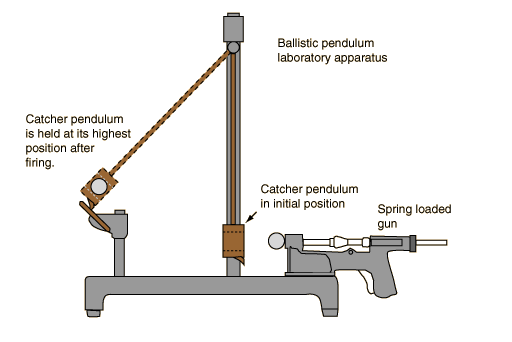In this lab you will study an inelastic collision using a Blackwood ballistic pendulum. The colliding bodies are a small metal ball, which is fired from a spring loaded gun, and a metal receptacle, or catcher. The receptacle is also the bob of a simple pendulum. Initially the pendulum is at rest. When the gun fires, the ball collides with the pendulum and is trapped in the catcher which then starts to swing. A ratchet and pawl system catches the pendulum at the height of its swing.

The best way to understand this experiment is to divide it into three separate events. First, the gun fires and the ball of mass m travels horizontally with initial velocity U In the absence of external forces, the horizontal component of its velocity will not change. The horizontal component of the ball's initial linear momentum is: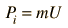(1)

In the second event, the ball collides with the "catcher" of mass M and is trapped by the spring. The system loses kinetic energy in the deformation of the spring and the creation of sound. Linear momentum, however, must be conserved. The pendulum, of mass (M + m), moves with a new horizontal velocity, V. The momentum of the system is now: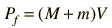(2)

Since the two momenta are equal, we can solve for U: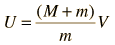(3)

Finally, the system acts like a simple pendulum. It moves upward and is caught by the ratchet at the highest part of its swing. The ratchet and pawl are designed to exert negligible force on the pendulum while it is moving upward, so mechanical energy is conserved. This means that the pendulum's kinetic energy at the bottom of its swing must equal its potential energy at the top of its swing. The change in height, h, of the center of mass can be easily measured. We can then solve for V: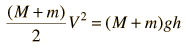(4)

Therefore: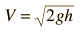(5)

Substitute this value for V into equation 3: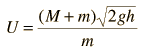(6)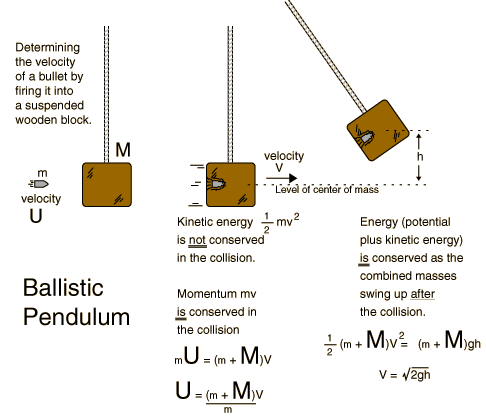There is a different, more direct way to measure the initial velocity of the ball. We can fire the gun horizontally from a known height and measure its range, R. The velocity, U, is given by: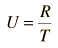(7)

where T is the time of flight. We can solve for the time of flight because the ball's horizontal and vertical motion are independent. As it moves horizontally, the ball also falls because of the influence of gravity. If the ball drops a distance Y: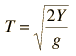(8)

solve for U: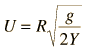(9)

Procedure

Use MKS units. Measure masses to 0.001 kg. Measure lengths to 0.001 m.

NEVER POINT THE GUN AT ANYONE !!!

Your instructor will show you how to load and fire the gun. Occasionally the ball will miss the catcher; therefore do not fire it if anyone is within range of the ball.

Be careful when you remove the ball from the catcher; push the spring inward to release the ball. The spring will break if you pull it backwards.

Part I

1. Unscrew the set screw that holds the pendulum at the top of the apparatus. Measure the mass of the ball (m) and of the ball and pendulum together (M + m). Reassemble the apparatus. Make sure that the pendulum can swing freely with a minimum of side-to-side motion.

2. Fire the gun and record the number of the notch in which the pendulum comes to rest. Repeat twice. If the notch numbers vary greatly, or if you have other problems, consult your instructor.

3. While the pendulum is hanging freely, measure the height of the center of mass marker from the base of the apparatus.

4. Average the notch numbers and raise the pendulum to that notch. Measure the height of the center of mass marker from the base of the apparatus. The difference between the two heights is h.

5. Use equation 6 to find the initial velocity of the ball.

Part II

6. Leave the pendulum raised. Position the apparatus near the end of the table so that it is aimed out across the floor with a range of about three meters. Shoot the ball and note where it hits the floor. Make sure that the gun does not move. Use masking tape to secure a piece of cork pad with a carbon set on it to the floor where the ball landed.

7. Fire the ball three more times or until you have three marks on the carbon set.

8. Put the gun back on the gun rod but do not cock it. Measure the distance from the bottom of the ball to the floor. This is Y. Also, measure the horizontal distance from the point on the floor directly below the ball to the center of the marks on the carbon set. This distance is R.

9. Use equation 9 to find U. Find the percent difference between the two values for U.

Questions

1. Only a fraction, F, of the ball's kinetic energy is transferred to the ball and catcher during the collision: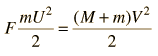(10)

With a bit of algebra you can show that: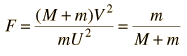(11)

What was the fraction of kinetic energy transferred during the collision?

2. Why was the distance Y measured from the bottom of the ball rather than the center of mass?

3. In part II, if the velocity of the ball had been doubled due to a stronger gun spring, how would the ball's time of flight have changed?

4. Which method of finding the velocity of the ball do you think was more accurate? Explain why.

The Ballistic Pendulum

Part I

mass of ball (m) __________

mass of ball and catcher (m + M) __________

notch 1 _____ notch 2 _____ notch 3 _____

average notch number _____

resting center of mass height _______________

raised center of mass heightĘ_______________

change in height __________

U = _______________

Part II

Y = _______________

R = _______________

U = _______________

% difference = _______________

Index
HyperPhysics***** Physics 7110 Go Back